# Vectorization with square point buffers

This year (2014) the buffer algorithm of Boost.Geometry was finally released in Boost 1.56

With buffer we mean a zone around a geometry. This is the GIS term, also used by OGC. In other environments it is often known as inflate / deflate, or shrink and expand, or Minkowski sum.

In GIS the buffer (with a positive distance) creates a larger version of e.g. a polygon. If a negative distance is specified, a smaller version is created.

As the documentation shows, various strategies can be used to:
• control the buffer-distance (either symmetric or asymmetric)
• control the joins (rounded or miter)
• control the ends for linestrings (rounded or flat)
• control the sides (straight)
• control the zones around points (or degenerated linestrings) (circular or square)
Strategies are provided, but they can also be provided by the library user, e.g. to create some fancy effects.

This short blog gives an example of the strategies for zones around points, using the square version. This strategy has the nice property that can be used to convert grids or images to vectors (polygons).

## strategy::buffer::point_square

See also the Wiki page. Pixels are converted to polygons. Suppose we add all centers of all filled pixels (e.g. the black pixels) into a multi point. We then buffer that multi-point with a distance of half the pixel size. And we use the strategy to create square buffers around each point. Then all adjacent gridcells will be connected. A polygon (or a multi-polygon) will be created, which is exactly what we wanted...

Suppose we have the following image (from this source) of a black cat.We create a multi-point of it, that is done manually:

```MULTIPOINT(1 0,2 0,3 0,4 0,5 0,6 0,7 0,   0 1,1 1,2 1,3 1,4 1,5 1,7 1,8 1,
0 2,1 2,2 2,3 2,4 2,5 2,8 2, 0 3,1 3,2 3,3 3,4 3,5 3,8 3,   0 4,1
4,2 4,3 4,4 4,5 4,8 4, 0 5,1 5,2 5,3 5,4 5,5 5,8 5,   1 6,2 6,3 6,4
6,7 6,8 6,   1 7,2 7,3 7,4 7,7 7, 1 8,2 8,3 8,4 8,7 8,   2 9,3
9,   6 9,7 9,   1 10,2 10,3 10,4 10,   1 11,2 11,3 11,4
11,   1 12,2 12,3 12,4 12,   1 13,4 13)```

If we use the next code fragment:

`    typedef boost::geometry::model::d2::point_xy<double> point;`
`    typedef boost::geometry::model::polygon<point> polygon;`
`    boost::geometry::strategy::buffer::distance_symmetric<double> distance_strategy(0.5);`
`    boost::geometry::strategy::buffer::join_round join_strategy;`
`    boost::geometry::strategy::buffer::end_round end_strategy;`
`    boost::geometry::strategy::buffer::point_square point_strategy;`
`    boost::geometry::strategy::buffer::side_straight side_strategy;`
`    boost::geometry::model::multi_polygon<polygon> result;`
`    boost::geometry::model::multi_point<point> mp;`
`    boost::geometry::read_wkt(cat, mp); // the cat std::string is somewhere defined`
`    boost::geometry::buffer(mp, result,`
`                distance_strategy, side_strategy,`
`                join_strategy, end_strategy, point_strategy);`

The resulting polygon will look like this:# Preludes in all 24 major and minor keys

Many composers in classical music have written sets of preludes in all major and minor, so 24, keys. The first well-known composer who wrote such a set was J.S. Bach, with his Well-Tempered Clavier. He wrote two sets, and each prelude is followed by a fuga. So 2*24*2=96 pieces. After him, at least 20 composers created similar sets (though often without fuga's) for piano, in all 24 keys. This blog links three sets, the prelude sets of Chopin, Scriabin and Kapustin.

#### Chopin

Chopin's well-known set is Opus 28, 24 preludes for piano solo. The preludes are in the order of the circle of fifths, with major and minor keys alternating. The preludes which are linked below are played by Arthur Rubinstein.

Interesting with this sequence is that all preludes in major are numbered odd, and all preludes in minor are numbered even. Also note that, because of this and the properties of the circle of fifths, the number of sharps/flats is equal for all pairs. So 1 and 2 have no sharps nor flats, 3 and 4 have one sharp, 5 and 6 have two, this is increasing until 13 and 14 (having either 6 flats or 6 sharps), then it switches to 5 flats for 15 and 16, this number is decreasing, until finally 23 and 24 have one flat.

#### Scriabin

Scriabin's set is Opus 11, 24 preludes for piano solo. He used exactly the same sequence as Chopin did. Number 5 was written in Amsterdam, in 1896. Number 8 is magnificently (and slowly) played by his friend Rachmaninov (!), here. Below are links to all preludes, played by Sofronitsky, a famous Scriabin interpreter and also his son-in-law. Scriabin started another set but did not finish that set. Preludes of this unfinished set are spread over Opus 13, 15, 16, and 17.

#### Kapustin

Kapustin's set is Opus 53, 24 preludes for piano solo. Kapustin used the same sequence as Chopin and Scriabin. These preludes are written in 1989, and denoted as in Jazz Style. Writing Jazz idioms in classical forms is Kapustin's speciality. Kapustin's set of preludes is marvelous. Below you find youtube links to all 24 preludes, played by the composer himself, Nikolai Kapustin. Besides this Opus 53, Kapustin wrote 20 piano sonata's, 6 piano concerto's, and much more. He is on the way to become the best componist of the 20th or 21th century! But still unknown to most people. Kapustin did also write another set of 24 preludes and fuga's in all keys, Opus 82. This set is not discussed here (because of similarity - it is more similar to Bach's Well-Tempered Clavier).

I could have added more sets, for example, I like for example Rachmaninov too. But the sets below are quite similar, using the same sequence, with composer names all ending with "in" :-), so I finished it this way. The images of the circles of fifths are a bit inspired on the Wiki source, but for the rest created from scratch for this blog page.

Enjoy the music, 72 preludes.

# SQLite, Ado.Net, SpatiaLite

SQLite is a small but great database. SpatiaLite is its spatial extension. ADO.NET is the method to access databases in .NET.

This blog shows how to combine them, because I did found all information on the web but not a complete and up-to-date story.

## SQLite and Ado.Net

An introduction (up and running in 3 minutes) is here. It is good but not up-to-date. In the meantime the ADO.NET driver is transferred from sourceforge/phxsoftware to sqlite.org, which is of course a good sign.

There is only one piece of software which you really need and that is the ADO.NET driver. Furthermore there are some optional (but very useful tools) which I describe below.

### ADO.NET driver

The current ADO.NET driver page is here, and more specificly you can download the drivers from this page. Download for example the sqlite-netFx40-setup-bundle-x86-2010-1.0.77.0.exe (10.25 MiB)  (at least I did) and install it. The installation folder will contain the driver, and also a manual and a sample database (northwindEF.db).

### Setting up the project

Create a new project (or use an existing) in C# Express or Visual Studio (I'm using 2010). I created a Console Project.

Most important is of course to add a reference. Typically you need  the System.Data.SQLite.dll from the installation folder (typically "c:\Program Files (x86)\System.Data.SQLite\2010\bin" ). You can either reference it from there or copy it to your project folder and reference it.

### Writing the code.

See also the original 3 minutes introduction for some code. Here a different sample is presented. I like complete code and I also include some extra optional pieces which I needed (creating a new database from scratch, and turning on foreign keys).

So a complete program looks like:
`// Copyright (c) 2011 Barend Gehrels using System; using System.Data.Common; using System.Data.SQLite; namespace SQLiteAndAdoDotNet {     class Program     {         const string mydb = @"c:\temp\mydatabase.db";         static void Main(string[] args)         {             bool isNew = false;            if (!System.IO.File.Exists(mydb))             {                 // Create a new SQLite database if it does not exist                SQLiteConnection.CreateFile(mydb);                 isNew = true;             }             using (SQLiteConnection connection = new SQLiteConnection(@"Data Source=" + mydb))             {                 connection.Open();                 // Recommended action for any SQLite database                ExecuteStatement("PRAGMA foreign_keys = ON", connection);                 if (isNew)                 {                     // Create a table for a new database                     ExecuteStatement(@"                         create table mytable                             (                                 id integer primary key autoincrement,                                 myfield text,                                 mydate integer -- dates can be stored as either text, real or integer                             )                         ", connection);                 }                 // Insert something                 ExecuteStatement("insert into mytable(myfield,mydate) values('my row',date('now'))",                     connection);                 // Retrieve something                 using (SQLiteCommand command = new SQLiteCommand("select *,datetime(mydate) as dt_ok from mytable",                             connection))                 {                     using (DbDataReader reader = command.ExecuteReader())                    {                         while (reader.Read())                         {                             System.Console.WriteLine(String.Format(                                 "Row id={0} field={1} date={2}, dt={3}",                                 reader["id"], reader["myfield"],                                 reader["mydate"], reader["dt_ok"]));                         }                     }                 }             }             System.Console.WriteLine("Done");         }         private static void ExecuteStatement(string statement, SQLiteConnection connection)        {             using (SQLiteCommand command = new SQLiteCommand(statement, connection))            {                 command.ExecuteNonQuery();             }         }     } } `

I factored out one method to avoid repetitions. In another blog I'll show you a different approach.

The foreign key pragma is of course not necessary in this short sample. But in a "real" database, with a datamodel and foreign keys, you will need it. Otherwise cascaded deletes will fail unexpectedly!

### One caveat

Beware for datetime fields, stored as real or integer! SQLite stores date types in any field, which is reasonable. But if you select such a date(time) field in the normal way you will get only the year back! So alas you have to cast it with the SQLite datetime function (described here). Dates stored as text do not have this caveat.

### Useful tools

I like the SQLite Studio which can be downloaded freely from here. You can hardly without such a tool and this one is really cool. If you are coming from SQL Server you might also like a converter, and this one is good, free, and with source.

## Using SpatialLite

Using SpatialLite is pretty much the same, from ADO.NET perspective. But of course you have to download some extra libraries. The SpatialLite library of course, but you also need at least two extra libraries. So here we go (see also StackOverflow):
• download the SpatiaLite DLL from this page (libspatialite-win-x86-2.3.1.zip)
• download proj-win as well from the same page (proj-win-x86-4.6.1.zip)
• download iconv as well from the same page (libiconv-win-x86-1.9.2.zip)
• download geos as well from the same page (geos-win-x86-3.1.1.zip) (I added geos later, after the comment of rmales, for me it was not necessary because it was found in my path, via PostGIS.)
But them all into your binary folder (Debug and Release). For our test project we also need a spatial database. You can create one from a shapefile (using the spatialite-gui from the same page) or download a test database from SpatialLite, here. I downloaded and extracted world.7z because I like to see world countries (see my other blogs).

Then, before you have to use it, you have to load the extension. That is pretty easy with an SQL statement, select load_extension('libspatialite-1.dll'). If it finds this DLL and the other two, it will run fine (and otherwise it will mention which is missing). Alas I could not get it working in sqlitestudio.

Then below is the program I used, and it runs fine. It is quite similar to the one above but I prefer to list it completely again. In next blog(s) we will do other interesting and maybe surprising things with it.
`// Copyright (c) 2011 Barend Gehrels using System; using System.Data.Common; using System.Data.SQLite; namespace SQLiteAndAdoDotNet {     class Program     {         const string mydb = @"c:\bdata\blogs\data\world.sqlite";         static void Main(string[] args)         {             using (SQLiteConnection connection = new SQLiteConnection(@"Data Source=" + mydb))             {                 connection.Open();                 // Recommended action for any SQLite database                ExecuteStatement("PRAGMA foreign_keys = ON", connection);                 // Required action for any SpatiaLite database                ExecuteStatement("select load_extension('libspatialite-1.dll')", connection);                 // Retrieve some cities in Europe                 using (SQLiteCommand command = new SQLiteCommand(                        @"                             select wup_aggl,AsText(Geometry) as wkt,                                X(Geometry) as x,Y(Geometry) as y                            from Cities                             where MBRContains(BuildMBR(-2,40,10,80),Geometry) = 1                         ", connection))                 {                     using (DbDataReader reader = command.ExecuteReader())                    {                         while (reader.Read())                         {                             System.Console.WriteLine(String.Format(                                 "City: {0} location: {1}, {2} wkt: {3}",                                 reader["wup_aggl"], reader["x"], reader["y"], reader["wkt"]));                         }                     }                 }             }             System.Console.WriteLine("Done");         }         private static void ExecuteStatement(string statement, SQLiteConnection connection)        {             using (SQLiteCommand command = new SQLiteCommand(statement, connection))            {                 command.ExecuteNonQuery();             }         }     } } `

### Useful tools

Of course you can use Quantum GIS to perfectly visualize your SpatiaLite database. The SpatiaLite page also contains many useful links.

## Summary

Above two complete programs to use SQLite and/or SpatiaLite in your .NET application. This is the C# version, VB.NET will work either.

# Linestring/polygon intersection

After a long while a blog again, a short one this time. I'm currently implementing intersections linestring/polygon for Boost.Geometry. Well, the basics (calculating intersection points) are already there for a long time, but for this combination the intersection points should be followed in another way and the correct pieces should be outputted.

When I do these things I normally check the results with both PostGIS and SQL Server. However, I'm encountering something weird in both of them.

## The testcase

My testcase is looking like this: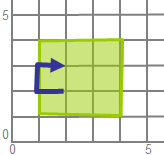## SQL Server

In SQL Server the intersection is correctly done, but the linestring is reversed! I'm using this query:
`with viewy as(   select      geometry::STGeomFromText('POLYGON((1 1,1 3,3 3,3 1,1 1))', 0) as p,      geometry::STGeomFromText('LINESTRING(2 2,1 2,1 3,2 3)', 0) as q)select   p.STIntersection(q).STLength() as len,   p.STIntersection(q).STAsText() as wktfrom viewy;`

and this is my output:

3    LINESTRING (2 3, 1 3, 1 2, 2 2)

You see, the linestring is reversed! It is not only reversed with this linestring, but with all linestrings. Even if they are, for example, complete inside the polygon. If I reverse the polygon (from clockwise to counter clockwise), the output is still reversed. Mysterious...

## PostGIS

Also a surprise in PostGIS.The query is looking there as following:

`with viewy as(   select      ST_GeomFromText('POLYGON((1 1,1 3,3 3,3 1,1 1))') as p,      ST_GeomFromText('LINESTRING(2 2,1 2,1 3,2 3)') as q)select    ST_Length(ST_Intersection(p, q)) as len,   ST_AsText(ST_Intersection(p, q)) as wkt from viewy;`
and this is here the result:

3;"MULTILINESTRING((1 2,1 3),(1 3,2 3),(2 2,1 2))"

I'm getting a multilinestring back! With respect to contents, it is OK. But it is surprising...

## OGC

I did not look it up but I don't think this specific behaviour is specified. So yes, all implementations will be correct but...

## Boost.Geometry

At the moment of writing, technically more difficult cases as this is one are not completely finished. But I can already tell that the linestring will not be reversed and that the output will consist of only one linestring...

# Spherical Side Formula

This blog describes an algorithm and a formula to determine the side of one point relative to a segment (two other points), all three points located on a sphere.

The side informarmation is necessary for Boost.Geometry. It is used, among others, in the within algorithm, to check if a point is inside a polygon (point in polygon). With the spherical version of side, it can be checked if a point on a sphere (the earth) is inside a spherical polygon (e.g. a polygon measured in latitude, longitude).

It is also known as orientation (see also here and here)

The Cartesian side is shown below. Point p is on the right side of segment p1-p2: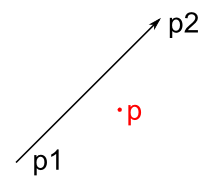A visualization of ths spherical side is shown below, also here, point p is right of segment p1-p2.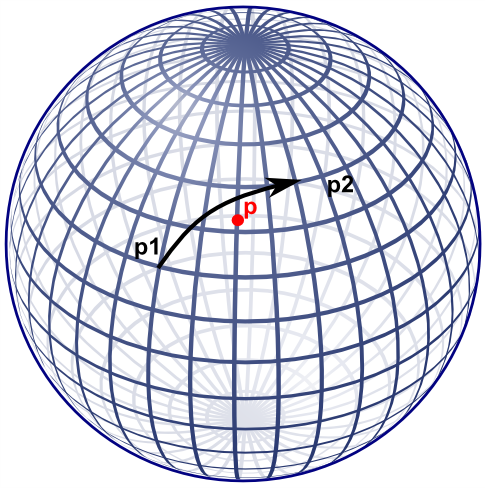Note that if the cartesian side formula would be used on lat-long coordinates, this specific point would result on the left side.

## Former approach

Boost.Geometry's former approach for spherical side used the useful aviation formulae of Williams, listed here. That did work but I was not completely satisfied about it:
• it takes two * (3 cos, 3 sin, 1 atan) for the course, and then another 2 sin, and 1 asin, so in total 17 goniometric functions
• it is non defined at the poles
• I don't understand it fully, it seemed to me that there should be an easier way
An obvious alternative is translate to 3D cartesian coordinates and why not? After working this out a bit, it appeared that it indeed requires less goniometric functions. These goniometric functions are less performant and less precise, so if they can be avoided, the better. Also the new approach is also relatively easy, from mathematical perspective.

## New approach

The new approach also uses sin and cos, but  less: for each of the points: 2 sin and 2 cos, in total 12 goniometric functions. Less then the former 17. More important, by design of Boost.Geometry, these might be precalculated such that for a spherical polygon it is not necessary to recalculate this again and again.

Besides that, the algorithm is easier to follow.

## The algorithm

Let us calculate a plane through the two points of the segment, and the center of the sphere. This is the plane also containing the Great Circle. Let us then calculate at which side of the plane the point is located, either left of the plane, or right of the plane, or on the plane itself. The side with respect to the plane is also the side of the point with respect to the segment on the sphere.

To calculate a plane, all three points are transformed from spherical coordinates (longitude, latitude) to cartesian coordinates (x, y, z). Described a.o. here with clear images. We can use the unit sphere, it is not necessary to take the radius of the Earth (or another sphere or sphere-like object) into account. Amsterdam, for example, then lies somewhere here: (0.614161755 0.042946374 0.788010754).

Converting can conveniently be done using Boost.Geometry's transform algorithm (if p1 is stored in cs::spherical_equatorial):

typedef model::point<coordinate_type, 3, cs::cartesian> point3d;
point3d c1, c2, c3;
transform(p1, c1);
transform(p2, c2);
transform(p, c3);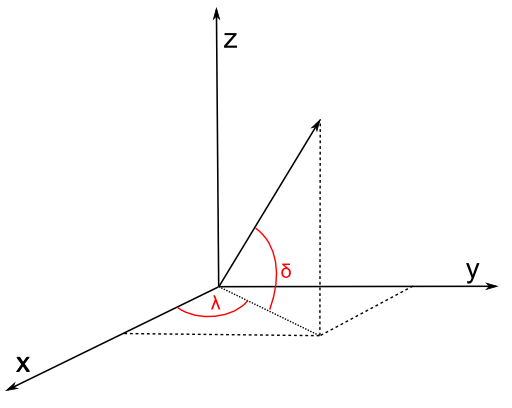Then we define a plane through the center of the Earth (0,0,0) and the two points. We use the generalized plane equation for that (a x + b y + c z + d = 0), described e.g. here. This is done by taking the cross product of the two vectors (a vector is here: a mathematical vector). These two vectors are normally called v and w. Because we use (0,0,0) the points are the vectors, we don't have to subtract anything to create a vector. So v is equivalent to c1, w is equivalent to c2.

The cross product is the normal vector, called n, normal to the plane, so perpendicular to the great circle of the two points. So we can take the cross product:

vector_type const n = geometry::cross_product(v, w);

We now have our generalized plane equation. To make that explicit, we can use the conventional symbols a, b, c:

coordinate_type const a = get<0>(n);
coordinate_type const b = get<1>(n);
coordinate_type const c = get<2>(n);

For the generalized plane equation, we should calculate d by subsituting one of the points going through the plane. Let us take (0,0,0)! By taking 0,0,0, all terms a x, b y, c z will be zero, so d is zero. Therefore we don't have to calculate d.

double const d = 0;

The generalized plane equation has the property that, if you substitute another point, it is either on the plane (then the result = 0), or at one side of the plane (then the result is positive), or at another side of the plane (then the result is negative). Just like the Cartesian side, described above. And that is exactly what we need:

distance_measure = a * get<0>(c3) + b * get<1>(c3) + c * get<2>(c3) + d;

and we have the result:

int side
= distance_measure > 0 ? 1 // left
: distance_measure < 0 ? -1 // right
: 0;

## Spherical Side Formula

We used convenient vector calculation here, making it a mathematical exercise. Of course we could write them all out and get a lot of goniometric functions back.

One big note here: geographers (as I am) always refer to coordinates on a sphere by defining 0 at the equator. But mathematicians define 0 at the upper pole of a sphere. Watch out, it is different. This blog assumes the geographic convention, so assumes a spherical equatorial coordinate system.

Let's present it as mathematic formulae (thanks to mathcast):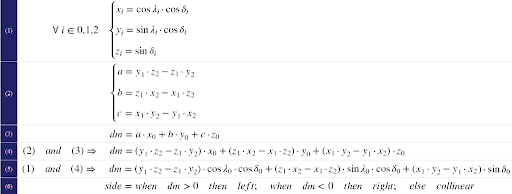The first formula converts to x,y,z. Be sure to enter all angles in radians. Also, be sure that λ is longitude (and comes first in Boost.Geometry) and δ is latitude (there are many conventions for this but I'm using them from here, and not from here and here and here). Latitude is 0 at the equator, 90 at the North Pole (again: mathematicians use 0 at the North Pole, 90 at the aquator; that is known as the spherical (polar) coordinate system, we now refer to the spherical equatorial coordinate system).

The second formula is the cross product and defines the terms of the generalized plane equation. The third formula calculates the distance measure (dm). Because (2) and (3) do not have repetitive clauses, they could be combined into (4) and then, if wished, again, with the conversion, into (5).

The sixth pseudo-formula then defines the result, it is an interpretation of the distance measure dm.

## Ellipsoidal Earth

The Earth is not spherical but approximates an ellipsoid, it is flattened at the poles. But the described spherical side formula works well on Earth. Let me try to prove that (note: I'm a geographer, not a mathematician).

A location defined by (λδ) essentially means a vector, either on a sphere, or on an ellipsoid. These vectors always have the same direction, so the same on a sphere as on an ellipsoid. Their lengths differ (on an ellipsoid they are often shorter) but the length of a vector does not influence the plane it encompasses. Therefore the plane formed by two locations and the center of the Earth is exactly the same plane as it is using a perfect sphere.

So: two points (a segment), on a sphere or on an ellipsoid, define the same plane.

The same applies for the third point. It is also a vector, having a direction. That vector, either on a sphere or on an ellipsoid, is located at the same side of the plane.

Q.E.D.

## Code

Because of the formulae above, I don't give (pseudo)code. It is quite easy. I created a small Excel sheet containing the formula is here. It contains this example (on this useful site): gcmap The formula is also in Boost.Geometry.

## Summary

Using some high-school mathematics I presented an algorithm and a formula to calculate at which side a point is with resepect to a segment, on a sphere or on the Earth. Google did not find this for me. So I hope that it will be of some use for some people. At least, it is useful for Boost.Geometry